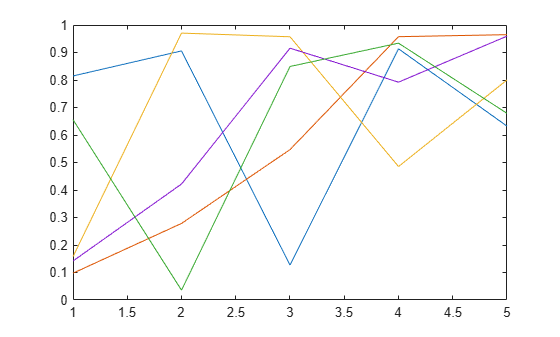# isgraphics

True for valid graphics object handles

## Syntax

``tf = isgraphics(H)``
``tf = isgraphics(H,type)``

## Description

example

````tf = isgraphics(H)` returns `true` for elements of `H` that are valid graphics objects and `false` where elements are not valid graphics objects or are graphics objects that have been deleted.```
````tf = isgraphics(H,type)` returns `true` for elements of `H` that are valid graphics objects of the type specified by the `type` argument. An object type is the value contained in the object’s `Type` property.```

## Examples

collapse all

Create a plot and return the handle array. Test array for valid handles.

`H = plot(rand(5));``isgraphics(H)`
```ans = 5x1 logical array 1 1 1 1 1 ```

Create a plot and return the handle array. Concatenate with other graphics objects and test for handles that are of type `Line`.

`H = plot(rand(5)); ````a = [H;gca;gcf]; isgraphics(a,'line')```
```ans = 7x1 logical array 1 1 1 1 1 0 0 ```

## Input Arguments

collapse all

Input variable or expression that evaluates to graphics object handles.

Object type, specified as a character vector or string scalar. An object’s type is the value of its `Type` property.

## Output Arguments

collapse all

Result, returned as a logical array. Values of `1` indicate elements of the input array that are valid, and values of `0` indicate elements that are invalid. The result is an empty array when the input array is empty.

## Version History

Introduced in R2014b